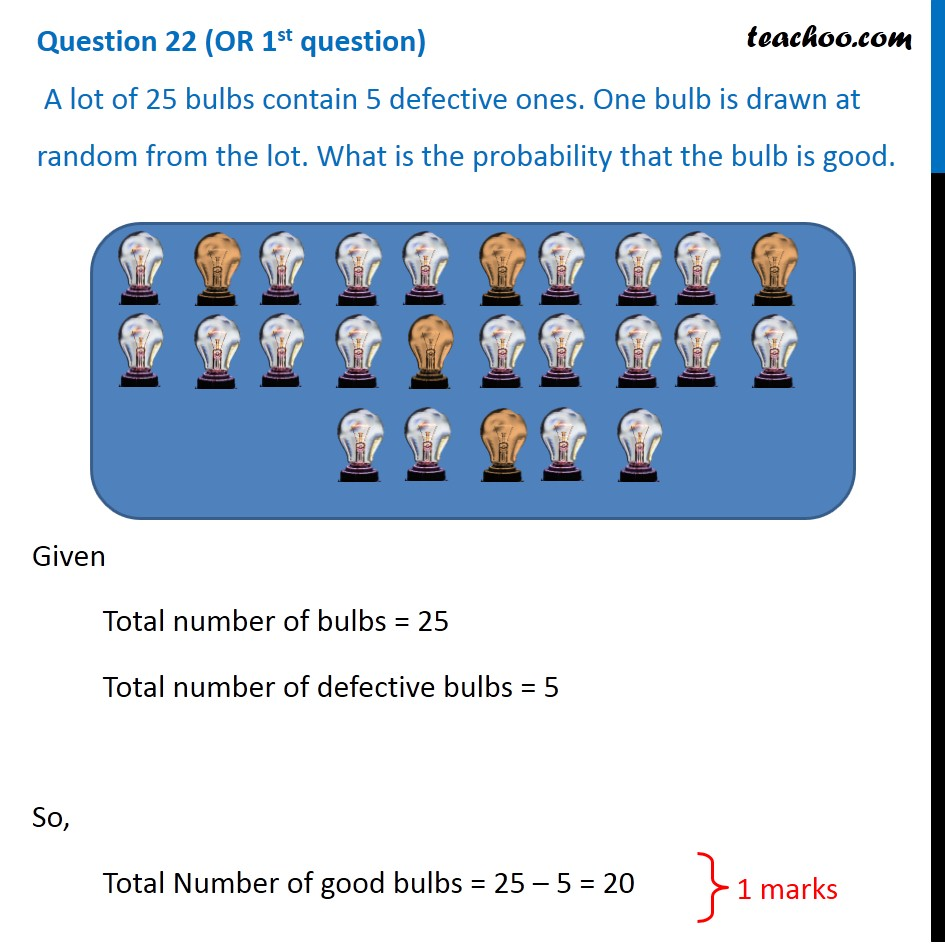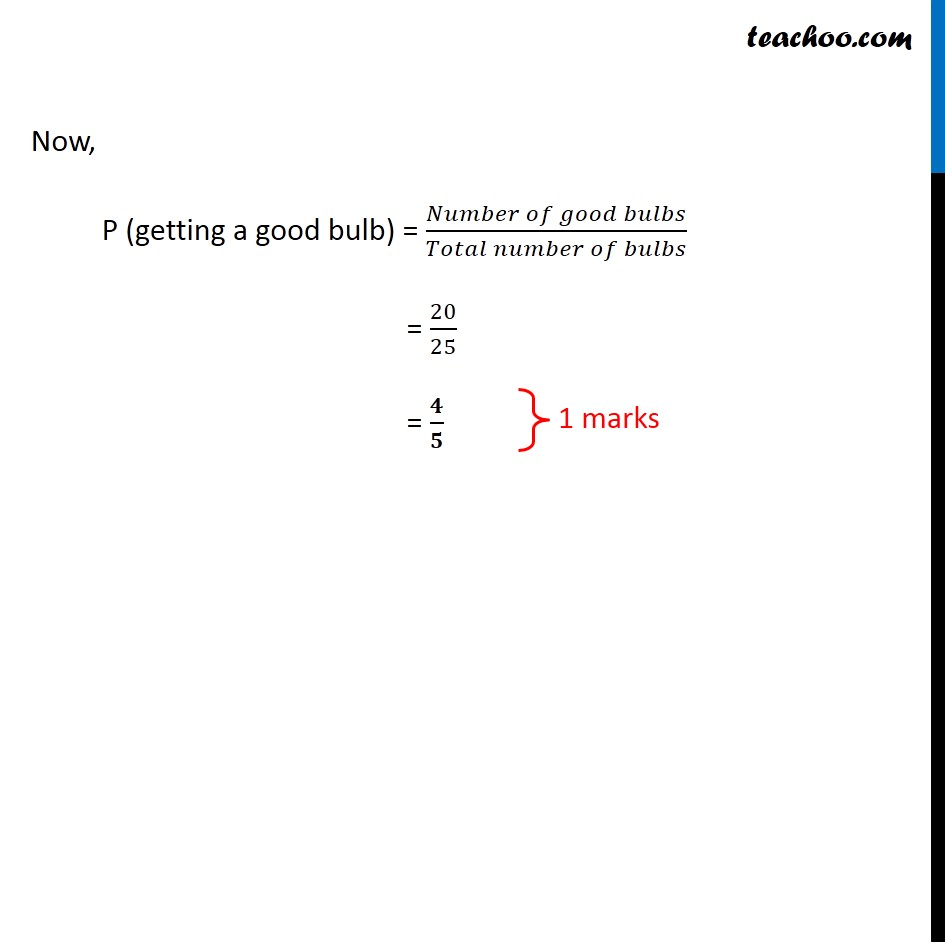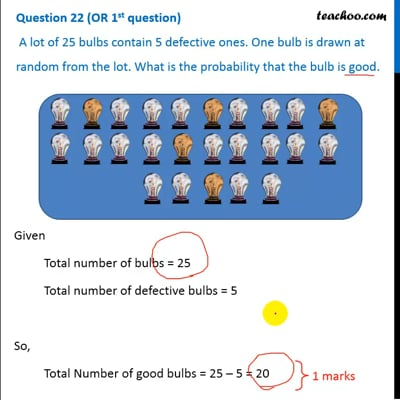CBSE Class 10 Sample Paper for 2020 Boards - Maths Basic

Class 10
Solutions of Sample Papers for Class 10 Boards

## A lot of 25 bulbs contain 5 defective ones. One bulb is drawn at random from the lot. What is the probability that the bulb is good.This video is only available for Teachoo black users

Note : This is similar to Ex 15.1, 17 of NCERT – Chapter 15 Class 10

### Transcript

Question 22 (OR 1st question) A lot of 25 bulbs contain 5 defective ones. One bulb is drawn at random from the lot. What is the probability that the bulb is good. Given Total number of bulbs = 25 Total number of defective bulbs = 5 So, Total Number of good bulbs = 25 – 5 = 20 Now, P (getting a good bulb) = (𝑁𝑢𝑚𝑏𝑒𝑟 𝑜𝑓 𝑔𝑜𝑜𝑑 𝑏𝑢𝑙𝑏𝑠)/(𝑇𝑜𝑡𝑎𝑙 𝑛𝑢𝑚𝑏𝑒𝑟 𝑜𝑓 𝑏𝑢𝑙𝑏𝑠) = 20/25 = 𝟒/𝟓